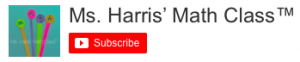# Multiplying Binomials Playlist

Our Multiplying Binomials Playlist is available on our YouTube Channel! There are two videos in this playlist:

1. Multiplying Simple Binomials
a) binomials with coefficients = 1.
2. Multiplying Complex Binomials
a) binomials with coefficients not equal to 1
b) binomials with 2 variables

Subscribe to the Ms. Harris’ Math Class YouTube channel to receive a notification when a new tutorial posts!-Ms. Harris